$$\require{cancel}$$

# 10.E: Fixed-Axis Rotation Introduction (Exercises)

•• Contributed by OpenStax
• General Physics at OpenStax CNX

## Conceptual Questions

### 10.1 Rotational Variables

1. A clock is mounted on the wall. As you look at it, what is the direction of the angular velocity vector of the second hand?
2. What is the value of the angular acceleration of the second hand of the clock on the wall?
3. A baseball bat is swung. Do all points on the bat have the same angular velocity? The same tangential speed?
4. The blades of a blender on a counter are rotating clockwise as you look into it from the top. If the blender is put to a greater speed what direction is the angular acceleration of the blades?

### 10.2 Rotation with Constant Angular Acceleration

1. If a rigid body has a constant angular acceleration, what is the functional form of the angular velocity in terms of the time variable?
2. If a rigid body has a constant angular acceleration, what is the functional form of the angular position?
3. If the angular acceleration of a rigid body is zero, what is the functional form of the angular velocity?
4. A massless tether with a masses tied to both ends rotates about a fixed axis through the center. Can the total acceleration of the tether/mass combination be zero if the angular velocity is constant?

### 10.3 Relating Angular and Translational Quantities

1. Explain why centripetal acceleration changes the direction of velocity in circular motion but not its magnitude.
2. In circular motion, a tangential acceleration can change the magnitude of the velocity but not its direction. Explain your answer.
3. Suppose a piece of food is on the edge of a rotating microwave oven plate. Does it experience nonzero tangential acceleration, centripetal acceleration, or both when: (a) the plate starts to spin faster? (b) The plate rotates at constant angular velocity? (c) The plate slows to a halt?

### 10.4 Moment of Inertia and Rotational Kinetic Energy

1. What if another planet the same size as Earth were put into orbit around the Sun along with Earth. Would the moment of inertia of the system increase, decrease, or stay the same?
2. A solid sphere is rotating about an axis through its center at a constant rotation rate. Another hollow sphere of the same mass and radius is rotating about its axis through the center at the same rotation rate. Which sphere has a greater rotational kinetic energy?

### 10.5 Calculating Moments of Inertia

1. If a child walks toward the center of a merry-go-round, does the moment of inertia increase or decrease?
2. A discus thrower rotates with a discus in his hand before letting it go. (a) How does his moment of inertia change after releasing the discus? (b) What would be a good approximation to use in calculating the moment of inertia of the discus thrower and discus?
3. Does increasing the number of blades on a propeller increase or decrease its moment of inertia, and why?
4. The moment of inertia of a long rod spun around an axis through one end perpendicular to its length is $$\frac{mL^{2}}{3}$$. Why is this moment of inertia greater than it would be if you spun a point mass m at the location of the center of mass of the rod (at $$\frac{L}{2}$$) (that would be $$\frac{mL^{2}}{4}$$)
5. Why is the moment of inertia of a hoop that has a mass M and a radius R greater than the moment of inertia of a disk that has the same mass and radius?

### 10.6 Torque

1. What three factors affect the torque created by a force relative to a specific pivot point?
2. Give an example in which a small force exerts a large torque. Give another example in which a large force exerts a small torque.
3. When reducing the mass of a racing bike, the greatest benefit is realized from reducing the mass of the tires and wheel rims. Why does this allow a racer to achieve greater accelerations than would an identical reduction in the mass of the bicycle’s frame?
4. Can a single force produce a zero torque?
5. Can a set of forces have a net torque that is zero and a net force that is not zero?
6. Can a set of forces have a net force that is zero and a net torque that is not zero?
7. In the expression $$\vec{r} \times \vec{F}$$ can $$|\vec{r}|$$ ever be less than the lever arm? Can it be equal to the lever arm?

### 10.7 Newton’s Second Law for Rotation

1. If you were to stop a spinning wheel with a constant force, where on the wheel would you apply the force to produce the maximum negative acceleration?
2. A rod is pivoted about one end. Two forces $$\vec{F}$$ and $$− \vec{F}$$ are applied to it. Under what circumstances will the rod not rotate?

## Problems

### 10.1 Rotational Variables

1. Calculate the angular velocity of Earth.
2. A track star runs a 400-m race on a 400-m circular track in 45 s. What is his angular velocity assuming a constant speed?
3. A wheel rotates at a constant rate of 2.0 x 103 rev/min. (a) What is its angular velocity in radians per second? (b) Through what angle does it turn in 10 s? Express the solution in radians and degrees.
4. A particle moves 3.0 m along a circle of radius 1.5 m. (a) Through what angle does it rotate? (b) If the particle makes this trip in 1.0 s at a constant speed, what is its angular velocity? (c) What is its acceleration?
5. A compact disc rotates at 500 rev/min. If the diameter of the disc is 120 mm, (a) what is the tangential speed of a point at the edge of the disc? (b) At a point halfway to the center of the disc?
6. Unreasonable results. The propeller of an aircraft is spinning at 10 rev/s when the pilot shuts off the engine. The propeller reduces its angular velocity at a constant 2.0 rad/s2 for a time period of 40 s. What is the rotation rate of the propeller in 40 s? Is this a reasonable situation?
7. A gyroscope slows from an initial rate of 32.0 rad/s at a rate of 0.700 rad/s2. How long does it take to come to rest?
8. On takeoff, the propellers on a UAV (unmanned aerial vehicle) increase their angular velocity for 3.0 s from rest at a rate of $$\omega$$ = (25.0t) rad/s where t is measured in seconds. (a) What is the instantaneous angular velocity of the propellers at t = 2.0 s? (b) What is the angular acceleration?
9. The angular position of a rod varies as 20.0t2 radians from time t = 0. The rod has two beads on it as shown in the following figure, one at 10 cm from the rotation axis and the other at 20 cm from the rotation axis. (a) What is the instantaneous angular velocity of the rod at t = 5 s? (b) What is the angular acceleration of the rod? (c) What are the tangential speeds of the beads at t = 5 s? (d) What are the tangential accelerations of the beads at t = 5 s? (e) What are the centripetal accelerations of the beads at t = 5 s?### 10.2 Rotation with Constant Angular Acceleration

1. A wheel has a constant angular acceleration of 5.0 rad/s2. Starting from rest, it turns through 300 rad. (a) What is its final angular velocity? (b) How much time elapses while it turns through the 300 radians?
2. During a 6.0-s time interval, a flywheel with a constant angular acceleration turns through 500 radians that acquire an angular velocity of 100 rad/s. (a) What is the angular velocity at the beginning of the 6.0 s? (b) What is the angular acceleration of the flywheel?
3. The angular velocity of a rotating rigid body increases from 500 to 1500 rev/min in 120 s. (a) What is the angular acceleration of the body? (b) Through what angle does it turn in this 120 s?
4. A flywheel slows from 600 to 400 rev/min while rotating through 40 revolutions. (a) What is the angular acceleration of the flywheel? (b) How much time elapses during the 40 revolutions?
5. A wheel 1.0 m in radius rotates with an angular acceleration of 4.0 rad/s2. (a) If the wheel’s initial angular velocity is 2.0 rad/s, what is its angular velocity after 10 s? (b) Through what angle does it rotate in the 10-s interval? (c) What are the tangential speed and acceleration of a point on the rim of the wheel at the end of the 10-s interval?
6. A vertical wheel with a diameter of 50 cm starts from rest and rotates with a constant angular acceleration of 5.0 rad/s2 around a fixed axis through its center counterclockwise. (a) Where is the point that is initially at the bottom of the wheel at t = 10 s? (b) What is the point’s linear acceleration at this instant?
7. A circular disk of radius 10 cm has a constant angular acceleration of 1.0 rad/s2; at t = 0 its angular velocity is 2.0 rad/s. (a) Determine the disk’s angular velocity at t = 5.0 s . (b) What is the angle it has rotated through during this time? (c) What is the tangential acceleration of a point on the disk at t = 5.0 s?
8. The angular velocity vs. time for a fan on a hovercraft is shown below. (a) What is the angle through which the fan blades rotate in the first 8 seconds? (b) Verify your result using the kinematic equations.1. A rod of length 20 cm has two beads attached to its ends. The rod with beads starts rotating from rest. If the beads are to have a tangential speed of 20 m/s in 7 s, what is the angular acceleration of the rod to achieve this?

### 10.3 Relating Angular and Translational Quantities

1. At its peak, a tornado is 60.0 m in diameter and carries 500 km/h winds. What is its angular velocity in revolutions per second?
2. A man stands on a merry-go-round that is rotating at 2.5 rad/s. If the coefficient of static friction between the man’s shoes and the merry-go-round is $$\mu$$S = 0.5, how far from the axis of rotation can he stand without sliding?
3. An ultracentrifuge accelerates from rest to 100,000 rpm in 2.00 min. (a) What is the average angular acceleration in rad/s2? (b) What is the tangential acceleration of a point 9.50 cm from the axis of rotation? (c) What is the centripetal acceleration in m/s2 and multiples of g of this point at full rpm? (d) What is the total distance traveled by a point 9.5 cm from the axis of rotation of the ultracentrifuge?
4. A wind turbine is rotating counterclockwise at 0.5 rev/s and slows to a stop in 10 s. Its blades are 20 m in length. (a) What is the angular acceleration of the turbine? (b) What is the centripetal acceleration of the tip of the blades at t = 0 s? (c) What is the magnitude and direction of the total linear acceleration of the tip of the blades at t = 0 s?
5. What is (a) the angular speed and (b) the linear speed of a point on Earth’s surface at latitude 30° N. Take the radius of the Earth to be 6309 km. (c) At what latitude would your linear speed be 10 m/s?
6. A child with mass 30 kg sits on the edge of a merrygo-round at a distance of 3.0 m from its axis of rotation. The merry-go-round accelerates from rest up to 0.4 rev/s in 10 s. If the coefficient of static friction between the child and the surface of the merry-go-round is 0.6, does the child fall off before 5 s?
7. A bicycle wheel with radius 0.3m rotates from rest to 3 rev/s in 5 s. What is the magnitude and direction of the total acceleration vector at the edge of the wheel at 1.0 s?
8. The angular velocity of a flywheel with radius 1.0 m varies according to $$\omega$$(t) = 2.0t. Plot ac(t) and at(t) from t = 0 to 3.0 s for r = 1.0 m. Analyze these results to explain when ac >> at and when ac << at for a point on the flywheel at a radius of 1.0 m.

### 10.4 Moment of Inertia and Rotational Kinetic Energy

1. A system of point particles is shown in the following figure. Each particle has mass 0.3 kg and they all lie in the same plane. (a) What is the moment of inertia of the system about the given axis? (b) If the system rotates at 5 rev/s, what is its rotational kinetic energy?1. (a) Calculate the rotational kinetic energy of Earth on its axis. (b) What is the rotational kinetic energy of Earth in its orbit around the Sun?
2. Calculate the rotational kinetic energy of a 12-kg motorcycle wheel if its angular velocity is 120 rad/s and its inner radius is 0.280 m and outer radius 0.330 m.
3. A baseball pitcher throws the ball in a motion where there is rotation of the forearm about the elbow joint as well as other movements. If the linear velocity of the ball relative to the elbow joint is 20.0 m/s at a distance of 0.480 m from the joint and the moment of inertia of the forearm is 0.500 kg-m2, what is the rotational kinetic energy of the forearm?
4. A diver goes into a somersault during a dive by tucking her limbs. If her rotational kinetic energy is 100 J and her moment of inertia in the tuck is 9.0 kg • m2, what is her rotational rate during the somersault?
5. An aircraft is coming in for a landing at 300 meters height when the propeller falls off. The aircraft is flying at 40.0 m/s horizontally. The propeller has a rotation rate of 20 rev/s, a moment of inertia of 70.0 kg • m2, and a mass of 200 kg. Neglect air resistance. (a) With what translational velocity does the propeller hit the ground? (b) What is the rotation rate of the propeller at impact?
6. If air resistance is present in the preceding problem and reduces the propeller’s rotational kinetic energy at impact by 30%, what is the propeller’s rotation rate at impact?
7. A neutron star of mass 2 x 1030 kg and radius 10 km rotates with a period of 0.02 seconds. What is its rotational kinetic energy?
8. An electric sander consisting of a rotating disk of mass 0.7 kg and radius 10 cm rotates at 15 rev/s. When applied to a rough wooden wall the rotation rate decreases by 20%. (a) What is the final rotational kinetic energy of the rotating disk? (b) How much has its rotational kinetic energy decreased?
9. A system consists of a disk of mass 2.0 kg and radius 50 cm upon which is mounted an annular cylinder of mass 1.0 kg with inner radius 20 cm and outer radius 30 cm (see below). The system rotates about an axis through the center of the disk and annular cylinder at 10 rev/s. (a) What is the moment of inertia of the system? (b) What is its rotational kinetic energy?### 10.5 Calculating Moments of Inertia

1. While punting a football, a kicker rotates his leg about the hip joint. The moment of inertia of the leg is 3.75 kg • m2 and its rotational kinetic energy is 175 J. (a) What is the angular velocity of the leg? (b) What is the velocity of tip of the punter’s shoe if it is 1.05 m from the hip joint?
2. Using the parallel axis theorem, what is the moment of inertia of the rod of mass m about the axis shown below?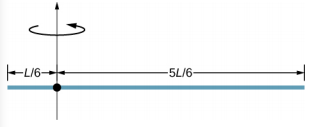1. Find the moment of inertia of the rod in the previous problem by direct integration.
2. A uniform rod of mass 1.0 kg and length 2.0 m is free to rotate about one end (see the following figure). If the rod is released from rest at an angle of 60° with respect to the horizontal, what is the speed of the tip of the rod as it passes the horizontal position?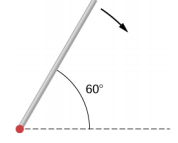1. A pendulum consists of a rod of mass 2 kg and length 1 m with a solid sphere at one end with mass 0.3 kg and radius 20 cm (see the following figure). If the pendulum is released from rest at an angle of 30°, what is the angular velocity at the lowest point?1. A solid sphere of radius 10 cm is allowed to rotate freely about an axis. The sphere is given a sharp blow so that its center of mass starts from the position shown in the following figure with speed 15 cm/s. What is the maximum angle that the diameter makes with the vertical?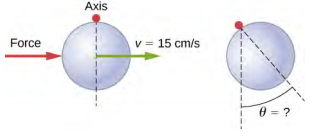1. Calculate the moment of inertia by direct integration of a thin rod of mass M and length L about an axis through the rod at L/3, as shown below. Check your answer with the parallel-axis theorem.### 10.6 Torque

1. Two flywheels of negligible mass and differ3ent radii are bonded together and rotate about a common axis (see below). The smaller flywheel of radius 30 cm has a cord that has a pulling force of 50 N on it. What pulling force needs to be applied to the cord connecting the larger flywheel of radius 50 cm such that the combination does not rotate?1. The cylinder head bolts on a car are to be tightened with a torque of 62.0 N·m. If a mechanic uses a wrench of length 20 cm, what perpendicular force must he exert on the end of the wrench to tighten a bolt correctly?
2. (a) When opening a door, you push on it perpendicularly with a force of 55.0 N at a distance of 0.850 m from the hinges. What torque are you exerting relative to the hinges? (b) Does it matter if you push at the same height as the hinges? There is only one pair of hinges.
3. When tightening a bolt, you push perpendicularly on a wrench with a force of 165 N at a distance of 0.140 m from the center of the bolt. How much torque are you exerting in newton-meters (relative to the center of the bolt)?
4. What hanging mass must be placed on the cord to keep the pulley from rotating (see the following figure)? The mass on the frictionless plane is 5.0 kg. The inner radius of the pulley is 20 cm and the outer radius is 30 cm.1. A simple pendulum consists of a massless tether 50 cm in length connected to a pivot and a small mass of 1.0 kg attached at the other end. What is the torque about the pivot when the pendulum makes an angle of 40° with respect to the vertical?
2. Calculate the torque about the z-axis that is out of the page at the origin in the following figure, given that F1 = 3 N, F2 = 2 N, F3 = 3 N, F4 = 1.8 N.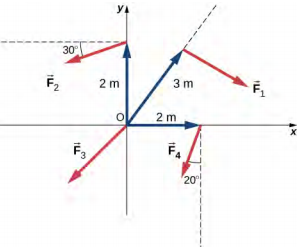1. A seesaw has length 10.0 m and uniform mass 10.0 kg and is resting at an angle of 30° with respect to the ground (see the following figure). The pivot is located at 6.0 m. What magnitude of force needs to be applied perpendicular to the seesaw at the raised end so as to allow the seesaw to barely start to rotate?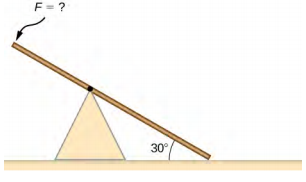1. A pendulum consists of a rod of mass 1 kg and length 1 m connected to a pivot with a solid sphere attached at the other end with mass 0.5 kg and radius 30 cm. What is the torque about the pivot when the pendulum makes an angle of 30° with respect to the vertical?
2. A torque of 5.00 x 103 N • m is required to raise a drawbridge (see the following figure). What is the tension necessary to produce this torque? Would it be easier to raise the drawbridge if the angle $$\theta$$ were larger or smaller?1. A horizontal beam of length 3 m and mass 2.0 kg has a mass of 1.0 kg and width 0.2 m sitting at the end of the beam (see the following figure). What is the torque of the system about the support at the wall?1. What force must be applied to end of a rod along the x-axis of length 2.0 m in order to produce a torque on the rod about the origin of 8.0$$\hat{k}$$ N • m?
2. What is the torque about the origin of the force (5.0 $$\hat{i}$$ − 2.0 $$\hat{j}$$ + 1.0$$\hat{k}$$) N if it is applied at the point whose position is: $$\vec{r}$$ = (−2.0 $$\hat{i}$$ + 4.0$$\hat{j}$$) m?

### 10.7 Newton’s Second Law for Rotation

1. You have a grindstone (a disk) that is 90.0 kg, has a 0.340-m radius, and is turning at 90.0 rpm, and you press a steel axe against it with a radial force of 20.0 N. (a) Assuming the kinetic coefficient of friction between steel and stone is 0.20, calculate the angular acceleration of the grindstone. (b) How many turns will the stone make before coming to rest?
2. Suppose you exert a force of 180 N tangential to a 0.280-m-radius, 75.0-kg grindstone (a solid disk). (a)What torque is exerted? (b) What is the angular acceleration assuming negligible opposing friction? (c) What is the angular acceleration if there is an opposing frictional force of 20.0 N exerted 1.50 cm from the axis?
3. A flywheel (I = 50 kg • m2) starting from rest acquires an angular velocity of 200.0 rad/s while subject to a constant torque from a motor for 5 s. (a) What is the angular acceleration of the flywheel? (b) What is the magnitude of the torque?
4. A constant torque is applied to a rigid body whose moment of inertia is 4.0 kg • m2 around the axis of rotation. If the wheel starts from rest and attains an angular velocity of 20.0 rad/s in 10.0 s, what is the applied torque?
5. A torque of 50.0 N • m is applied to a grinding wheel (I = 20.0 kg • m2) for 20 s. (a) If it starts from rest, what is the angular velocity of the grinding wheel after the torque is removed? (b) Through what angle does the wheel move through while the torque is applied?
6. A flywheel (I = 100.0 kg • m2) rotating at 500.0 rev/ min is brought to rest by friction in 2.0 min. What is the frictional torque on the flywheel?
7. A uniform cylindrical grinding wheel of mass 50.0 kg and diameter 1.0 m is turned on by an electric motor. The friction in the bearings is negligible. (a) What torque must be applied to the wheel to bring it from rest to 120 rev/min in 20 revolutions? (b) A tool whose coefficient of kinetic friction with the wheel is 0.60 is pressed perpendicularly against the wheel with a force of 40.0 N. What torque must be supplied by the motor to keep the wheel rotating at a constant angular velocity?
8. Suppose when Earth was created, it was not rotating. However, after the application of a uniform torque after 6 days, it was rotating at 1 rev/day. (a) What was the angular acceleration during the 6 days? (b) What torque was applied to Earth during this period? (c) What force tangent to Earth at its equator would produce this torque?
9. A pulley of moment of inertia 2.0 kg • m2 is mounted on a wall as shown in the following figure. Light strings are wrapped around two circumferences of the pulley and weights are attached. What are (a) the angular acceleration of the pulley and (b) the linear acceleration of the weights? Assume the following data: r1 = 50 cm, r2 = 20 cm, m1 = 1.0 kg, m2 = 2.0 kg.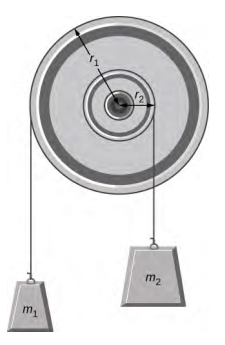1. A block of mass 3 kg slides down an inclined plane at an angle of 45° with a massless tether attached to a pulley with mass 1 kg and radius 0.5 m at the top of the incline (see the following figure). The pulley can be approximated as a disk. The coefficient of kinetic friction on the plane is 0.4. What is the acceleration of the block?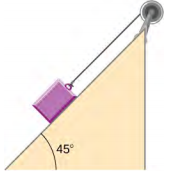1. The cart shown below moves across the table top as the block falls. What is the acceleration of the cart? Neglect friction and assume the following data: m1 = 2.0 kg, m2 = 4.0 kg, I = 0.4 kg • m2, r = 20 cm.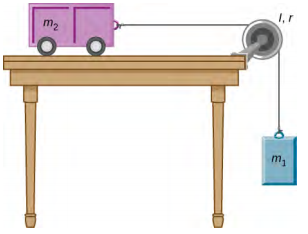1. A uniform rod of mass and length is held vertically by two strings of negligible mass, as shown below. (a) Immediately after the string is cut, what is the linear acceleration of the free end of the stick? (b) Of the middle of the stick?1. A thin stick of mass 0.2 kg and length L = 0.5 m is attached to the rim of a metal disk of mass M = 2.0 kg and radius R = 0.3 m. The stick is free to rotate around a horizontal axis through its other end (see the following figure). (a) If the combination is released with the stick horizontal, what is the speed of the center of the disk when the stick is vertical? (b) What is the acceleration of the center of the disk at the instant the stick is released? (c) At the instant the stick passes through the vertical?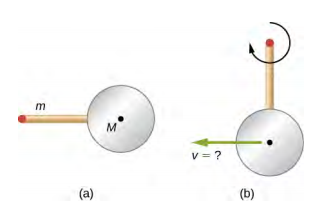### 10.8 Work and Power for Rotational Motion

1. A wind turbine rotates at 20 rev/min. If its power output is 2.0 MW, what is the torque produced on the turbine from the wind?
2. A clay cylinder of radius 20 cm on a potter’s wheel spins at a constant rate of 10 rev/s. The potter applies a force of 10 N to the clay with his hands where the coefficient of friction is 0.1 between his hands and the clay. What is the power that the potter has to deliver to the wheel to keep it rotating at this constant rate?
3. A uniform cylindrical grindstone has a mass of 10 kg and a radius of 12 cm. (a) What is the rotational kinetic energy of the grindstone when it is rotating at 1.5 x 103 rev/min? (b) After the grindstone’s motor is turned off, a knife blade is pressed against the outer edge of the grindstone with a perpendicular force of 5.0 N. The coefficient of kinetic friction between the grindstone and the blade is 0.80. Use the work energy theorem to determine how many turns the grindstone makes before it stops.
4. A uniform disk of mass 500 kg and radius 0.25 m is mounted on frictionless bearings so it can rotate freely around a vertical axis through its center (see the following figure). A cord is wrapped around the rim of the disk and pulled with a force of 10 N. (a) How much work has the force done at the instant the disk has completed three revolutions, starting from rest? (b) Determine the torque due to the force, then calculate the work done by this torque at the instant the disk has completed three revolutions? (c) What is the angular velocity at that instant? (d) What is the power output of the force at that instant?1. A propeller is accelerated from rest to an angular velocity of 1000 rev/min over a period of 6.0 seconds by a constant torque of 2.0 x 103 N • m. (a) What is the moment of inertia of the propeller? (b) What power is being provided to the propeller 3.0 s after it starts rotating?
2. A sphere of mass 1.0 kg and radius 0.5 m is attached to the end of a massless rod of length 3.0 m. The rod rotates about an axis that is at the opposite end of the sphere (see below). The system rotates horizontally about the axis at a constant 400 rev/min. After rotating at this angular speed in a vacuum, air resistance is introduced and provides a force 0.15 N on the sphere opposite to the direction of motion. What is the power provided by air resistance to the system 100.0 s after air resistance is introduced?1. A uniform rod of length L and mass M is held vertically with one end resting on the floor as shown below. When the rod is released, it rotates around its lower end until it hits the floor. Assuming the lower end of the rod does not slip, what is the linear velocity of the upper end when it hits the floor?1. An athlete in a gym applies a constant force of 50 N to the pedals of a bicycle at a rate of the pedals moving 60 rev/min. The length of the pedal arms is 30 cm. What is the power delivered to the bicycle by the athlete?
2. A 2-kg block on a frictionless inclined plane at 40° has a cord attached to a pulley of mass 1 kg and radius 20 cm (see the following figure). (a) What is the acceleration of the block down the plane? (b) What is the work done by the cord on the pulley?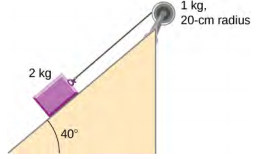1. Small bodies of mass m1 and m2 are attached to opposite ends of a thin rigid rod of length L and mass M. The rod is mounted so that it is free to rotate in a horizontal plane around a vertical axis (see below). What distance d from m1 should the rotational axis be so that a minimum amount of work is required to set the rod rotating at an angular velocity $$\omega$$?1. A cyclist is riding such that the wheels of the bicycle have a rotation rate of 3.0 rev/s. If the cyclist brakes such that the rotation rate of the wheels decrease at a rate of 0.3 rev/s2, how long does it take for the cyclist to come to a complete stop?
2. Calculate the angular velocity of the orbital motion of Earth around the Sun.
3. A phonograph turntable rotating at 33$$\frac{1}{3}$$ rev/min slows down and stops in 1.0 min. (a) What is the turntable’s angular acceleration assuming it is constant? (b) How many revolutions does the turntable make while stopping?
4. With the aid of a string, a gyroscope is accelerated from rest to 32 rad/s in 0.40 s under a constant angular acceleration. (a) What is its angular acceleration in rad/s2? (b) How many revolutions does it go through in the process?
5. Suppose a piece of dust has fallen on a CD. If the spin rate of the CD is 500 rpm, and the piece of dust is 4.3 cm from the center, what is the total distance traveled by the dust in 3 minutes? (Ignore accelerations due to getting the CD rotating.)
6. A system of point particles is rotating about a fixed axis at 4 rev/s. The particles are fixed with respect to each other. The masses and distances to the axis of the point particles are m1 = 0.1 kg, r1 = 0.2 m, m2 = 0.05 kg, r2 = 0.4 m, m3 = 0.5 kg, r3 = 0.01 m. (a) What is the moment of inertia of the system? (b) What is the rotational kinetic energy of the system?
7. Calculate the moment of inertia of a skater given the following information. (a) The 60.0-kg skater is approximated as a cylinder that has a 0.110-m radius. (b) The skater with arms extended is approximated by a cylinder that is 52.5 kg, has a 0.110-m radius, and has two 0.900-m-long arms which are 3.75 kg each and extend straight out from the cylinder like rods rotated about their ends.
8. A stick of length 1.0 m and mass 6.0 kg is free to rotate about a horizontal axis through the center. Small bodies of masses 4.0 and 2.0 kg are attached to its two ends (see the following figure). The stick is released from the horizontal position. What is the angular velocity of the stick when it swings through the vertical?1. A pendulum consists of a rod of length 2 m and mass 3 kg with a solid sphere of mass 1 kg and radius 0.3 m attached at one end. The axis of rotation is as shown below. What is the angular velocity of the pendulum at its lowest point if it is released from rest at an angle of 30°?1. Calculate the torque of the 40-N force around the axis through O and perpendicular to the plane of the page as shown below.1. Two children push on opposite sides of a door during play. Both push horizontally and perpendicular to the door. One child pushes with a force of 17.5 N at a distance of 0.600 m from the hinges, and the second child pushes at a distance of 0.450 m. What force must the second child exert to keep the door from moving? Assume friction is negligible.
2. The force of 20 $$\hat{j}$$ N is applied at $$\vec{r}$$ = (4.0 $$\hat{i}$$ − 2.0 $$\hat{j}$$) m. What is the torque of this force about the origin? 119. An automobile engine can produce 200 N • m of torque. Calculate the angular acceleration produced if 95.0% of this torque is applied to the drive shaft, axle, and rear wheels of a car, given the following information. The car is suspended so that the wheels can turn freely. Each wheel acts like a 15.0-kg disk that has a 0.180-m radius. The walls of each tire act like a 2.00-kg annular ring that has inside radius of 0.180 m and outside radius of 0.320 m. The tread of each tire acts like a 10.0-kg hoop of radius 0.330 m. The 14.0-kg axle acts like a rod that has a 2.00-cm radius. The 30.0-kg drive shaft acts like a rod that has a 3.20-cm radius.
3. A grindstone with a mass of 50 kg and radius 0.8 m maintains a constant rotation rate of 4.0 rev/s by a motor while a knife is pressed against the edge with a force of 5.0 N. The coefficient of kinetic friction between the grindstone and the blade is 0.8. What is the power provided by the motor to keep the grindstone at the constant rotation rate?

## Challenge Problems

1. The angular acceleration of a rotating rigid body is given by $$\alpha$$ = (2.0 − 3.0t) rad/s2. If the body starts rotating from rest at t = 0, (a) what is the angular velocity? (b) Angular position? (c) What angle does it rotate through in 10 s? (d) Where does the vector perpendicular to the axis of rotation indicating 0° at t = 0 lie at t = 10 s?
2. Earth’s day has increased by 0.002 s in the last century. If this increase in Earth’s period is constant, how long will it take for Earth to come to rest?
3. A disk of mass m, radius R, and area A has a surface mass density $$\sigma = \frac{mr}{AR}$$ (see the following figure). What is the moment of inertia of the disk about an axis through the center?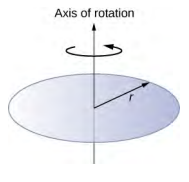1. Zorch, an archenemy of Rotation Man, decides to slow Earth’s rotation to once per 28.0 h by exerting an opposing force at and parallel to the equator. Rotation Man is not immediately concerned, because he knows Zorch can only exert a force of 4.00 x 107 N (a little greater than a Saturn V rocket’s thrust). How long must Zorch push with this force to accomplish his goal? (This period gives Rotation Man time to devote to other villains.)
2. A cord is wrapped around the rim of a solid cylinder of radius 0.25 m, and a constant force of 40 N is exerted on the cord shown, as shown in the following figure. The cylinder is mounted on frictionless bearings, and its moment of inertia is 6.0 kg • m2. (a) Use the work energy theorem to calculate the angular velocity of the cylinder after 5.0 m of cord have been removed. (b) If the 40-N force is replaced by a 40-N weight, what is the angular velocity of the cylinder after 5.0 m of cord have unwound?## Contributors

Samuel J. Ling (Truman State University), Jeff Sanny (Loyola Marymount University), and Bill Moebs with many contributing authors. This work is licensed by OpenStax University Physics under a Creative Commons Attribution License (by 4.0).